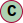# Class Humidity

3D vector sensor value with accuracy and timestamp.

#### Hierarchy-Diagram##### Legendclass

## Properties

accuracy: Accuracy<Unit, number | Vector3>
isVector3: true
timestamp: number
unit: Unit
x: number
y: number
z: number

## Methods

• Adds v to this vector.

#### Returns Humidity

• Sets this vector to a + b.

#### Returns Humidity

• Copies value of v to this vector.

#### Returns Humidity

• Sets this vector to cross product of itself and v.

#### Returns Humidity

• Sets this vector to cross product of a and b.

#### Returns Humidity

• Computes distance of this vector to v.

• ### Deprecated

#### Returns number

• Computes squared distance of this vector to v.

#### Returns Humidity

• Divides this vector by scalar s. Set vector to ( 0, 0, 0 ) if s == 0.

#### Returns Humidity

• Computes dot product of this vector and v.

#### Returns number

• Checks for strict equality of this vector and v.

• #### Returns Humidity

• Sets this vector's x, y and z value from the provided array or array-like.

#### Parameters

• ##### array: number[] | ArrayLike<number>

the source array or array-like.

• ##### `Optional` offset: number

(optional) offset into the array. Default is 0.

#### Returns number

• Computes length of this vector.

#### Returns number

• Computes squared length of this vector.

#### Returns Humidity

• Computes the Manhattan length (distance) from this vector to the given vector v

#### Returns number

• Computes the Manhattan length of this vector.

#### Returns Humidity

• Multiplies this vector by scalar s.

#### Returns Humidity

• Inverts this vector.

#### Returns Humidity

• Normalizes this vector.

#### Returns Humidity

• Sets this vector's x, y and z from Math.random

• #### Returns Humidity

• Sets value of this vector.

#### Returns Humidity

• Set the accuracy of the absolute position

### Returns

instance

#### Parameters

• ##### accuracy: number | Accuracy<Unit, number | Vector3>

Accuracy object or number

#### Returns Humidity

• Sets this vector's x, y and z components from the x, y, and z components of the specified Euler Angle.

#### Returns Humidity

• Normalizes this vector and multiplies it by l.

#### Returns Humidity

• Sets all values of this vector.

#### Returns Humidity

• Sets x value of this vector.

#### Returns Vector3

• Sets y value of this vector.

#### Returns Vector3

• Sets z value of this vector.

#### Returns Vector3

• Subtracts v from this vector.

#### Returns Humidity

• Sets this vector to a - b.

#### Returns Humidity

• Returns an array [x, y, z], or copies x, y and z into the provided array.

### Returns

The created or provided array.

#### Parameters

• ##### `Optional` array: number[]

(optional) array to store the vector to. If this is not provided, a new array will be created.

• ##### `Optional` offset: number

(optional) optional offset into the array.

#### Returns Vector3Tuple

• Copies x, y and z into the provided array-like.

### Returns

The provided array-like.

#### Parameters

• ##### array: ArrayLike<number>

array-like to store the vector to.

• ##### `Optional` offset: number

(optional) optional offset into the array-like.

#### Parameters

• ##### this: (new (...args: any[]) => T)
• new (...args: any[]): T

#### Parameters

• ##### this: (new (...args: any[]) => T)
• new (...args: any[]): T